Inverse Proportion

Chapter 13 Class 8 Direct and Inverse Proportions
Concept wise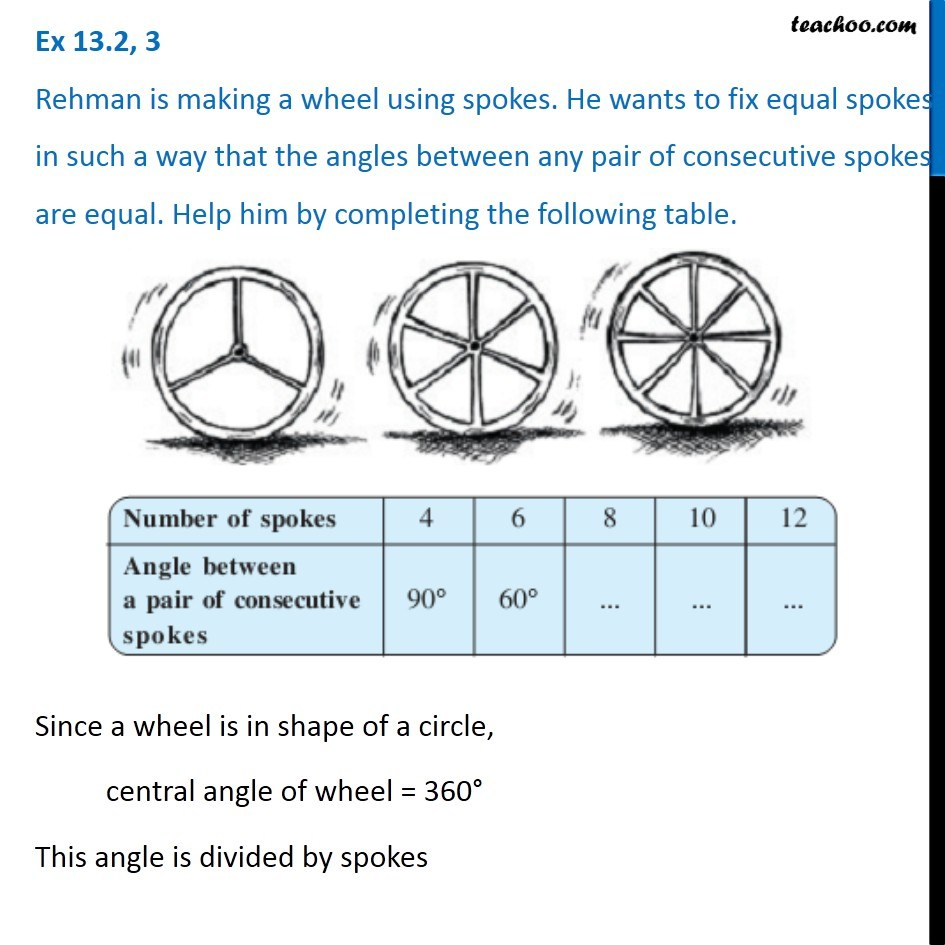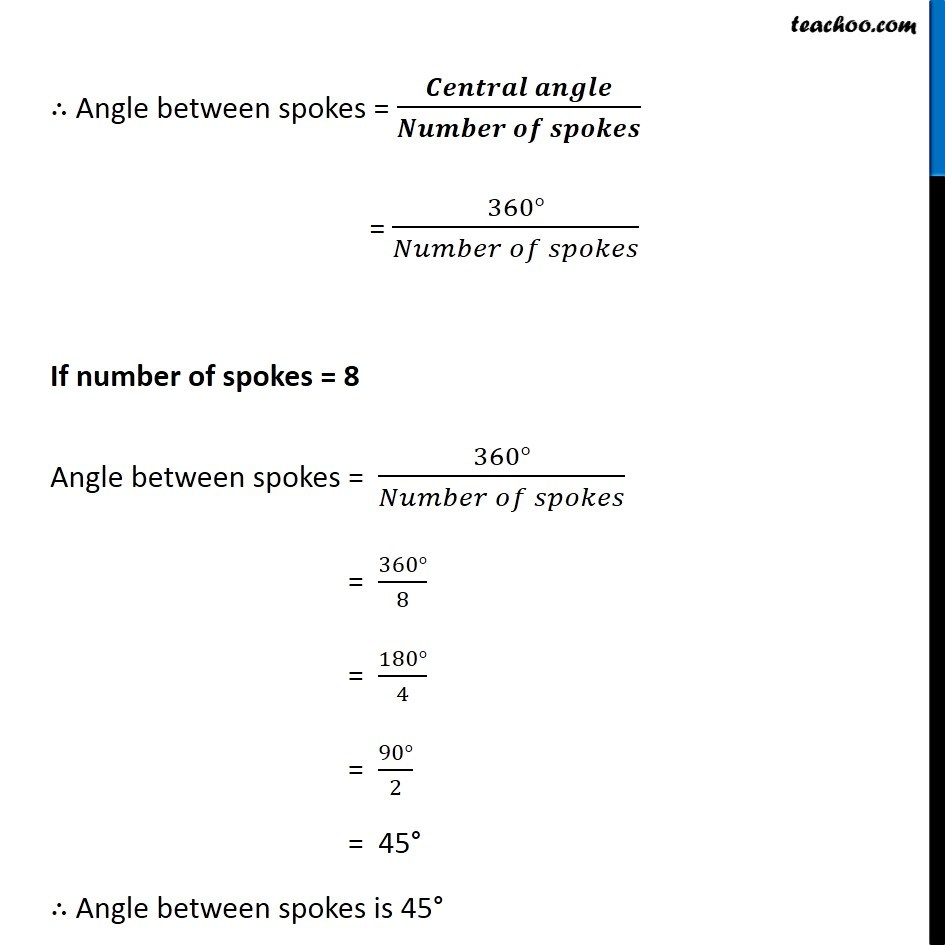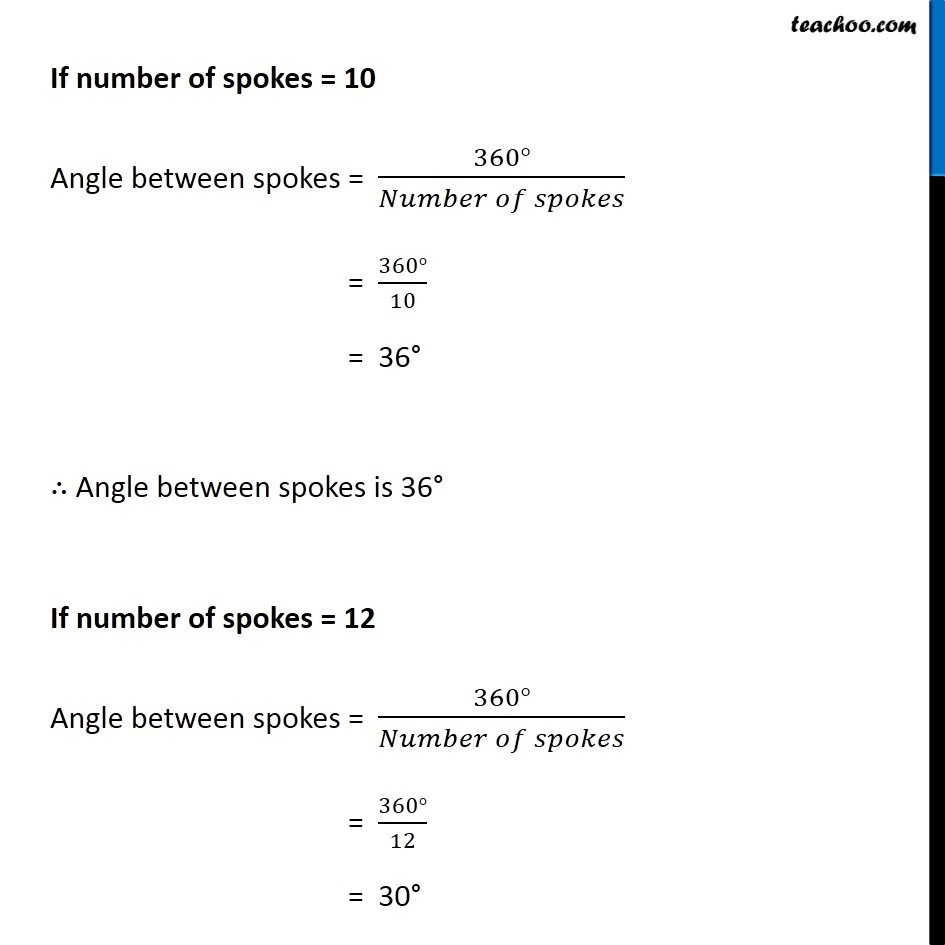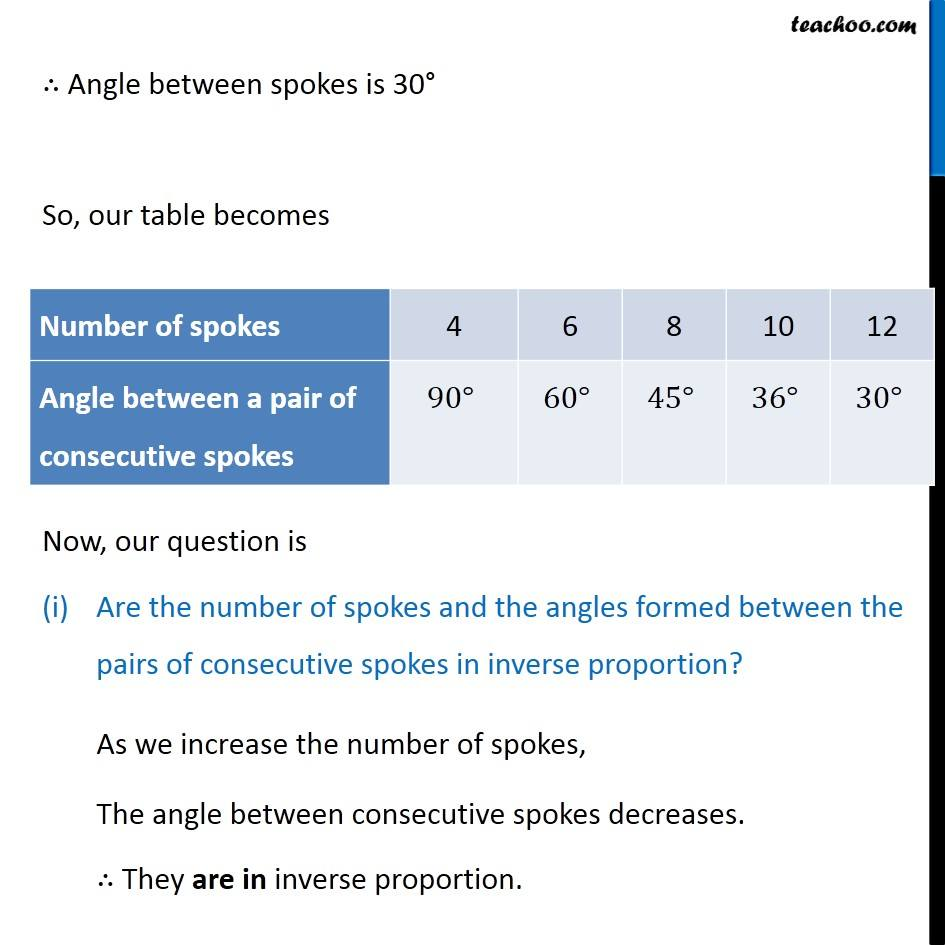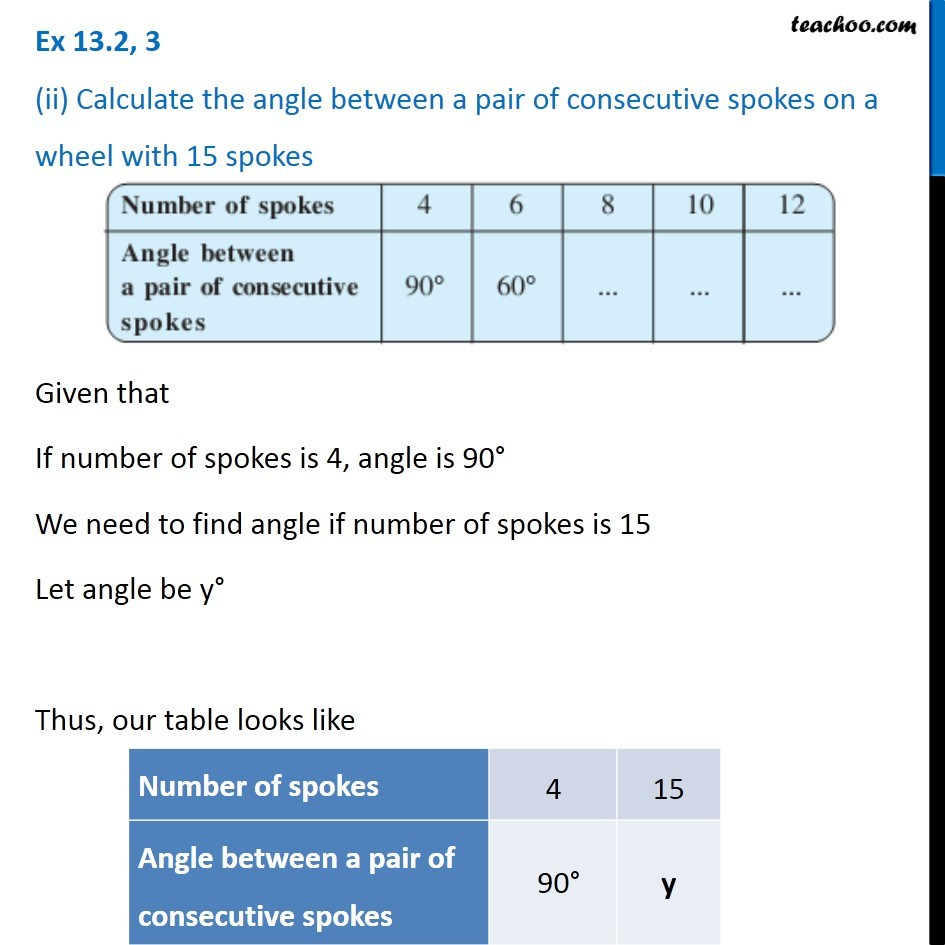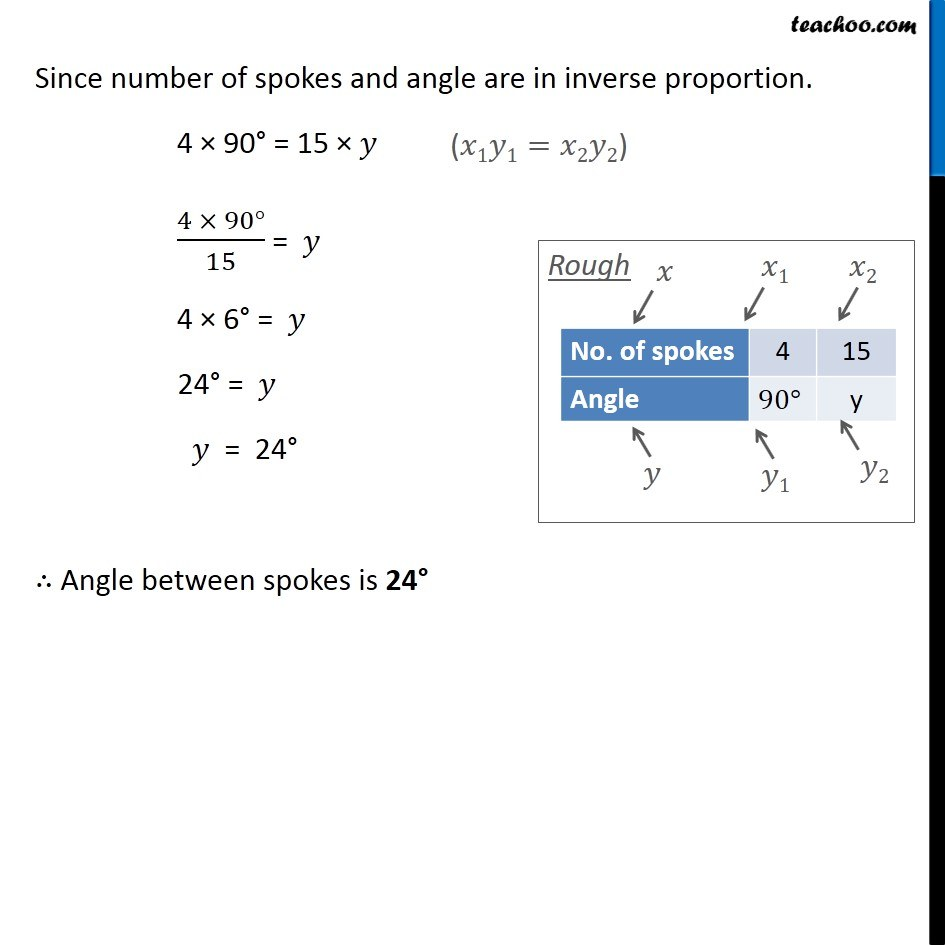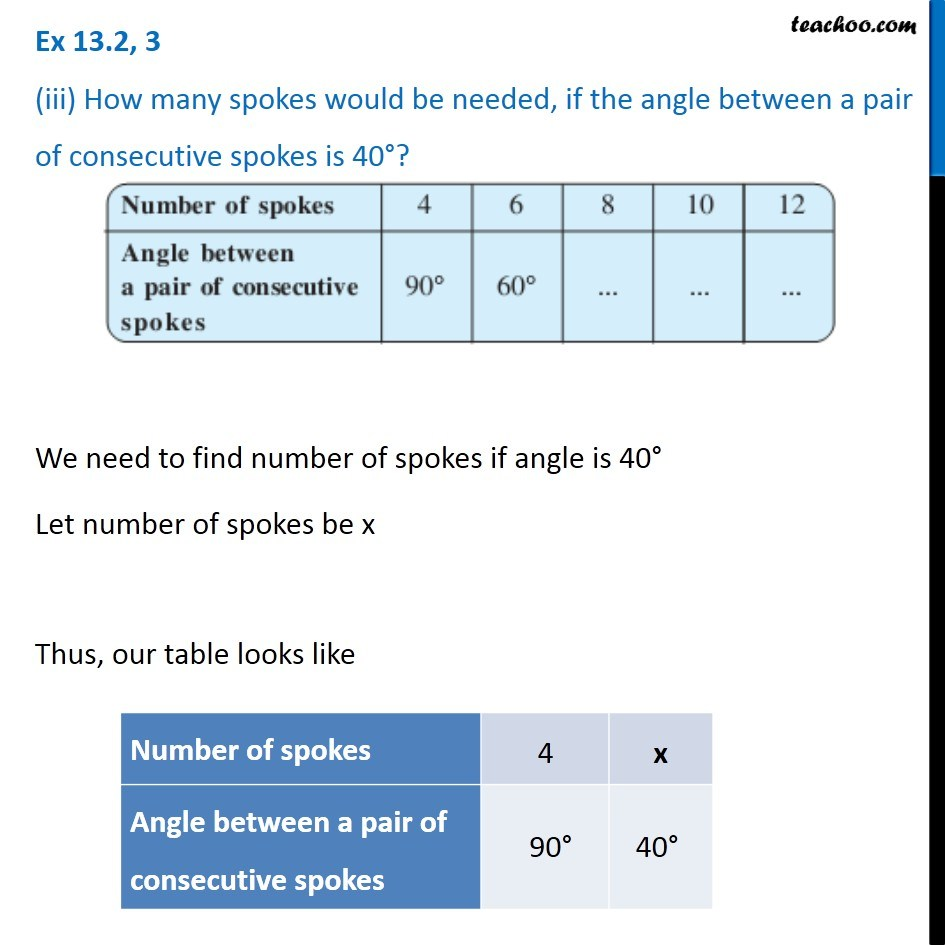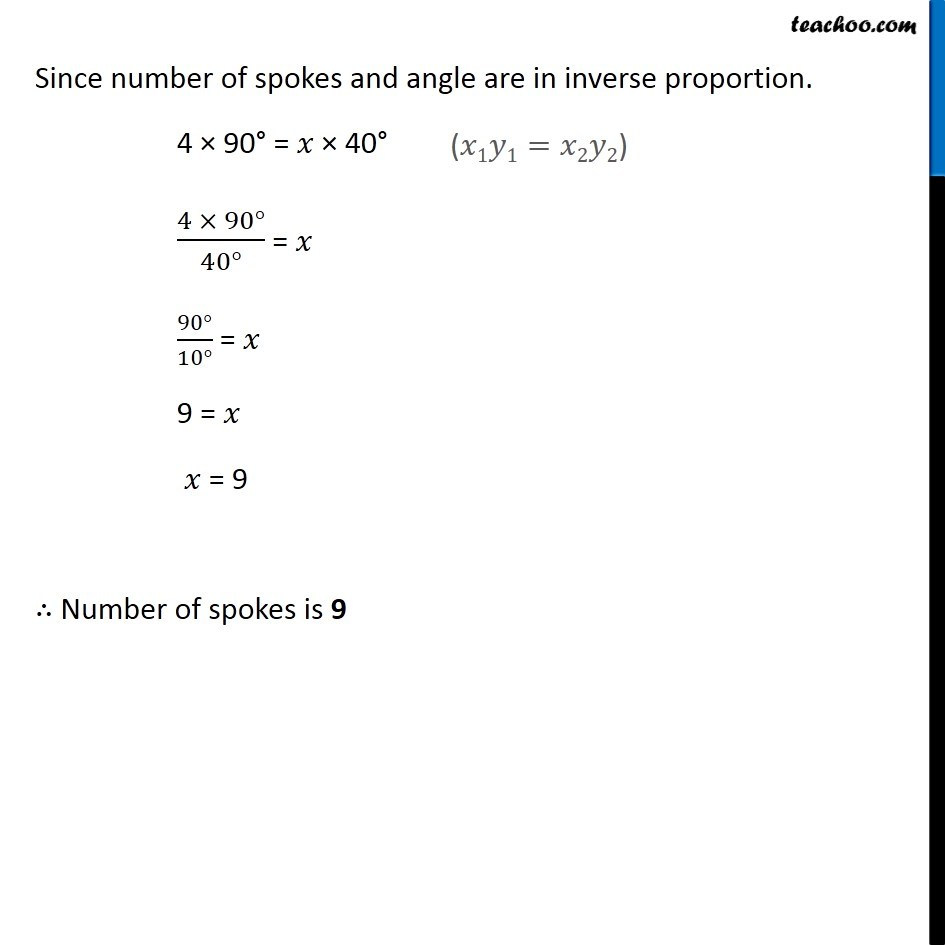### Transcript

Ex 13.2, 3 Rehman is making a wheel using spokes. He wants to fix equal spokes in such a way that the angles between any pair of consecutive spokes are equal. Help him by completing the following table. Since a wheel is in shape of a circle, central angle of wheel = 360° This angle is divided by spokes ∴ Angle between spokes = (𝑪𝒆𝒏𝒕𝒓𝒂𝒍 𝒂𝒏𝒈𝒍𝒆)/(𝑵𝒖𝒎𝒃𝒆𝒓 𝒐𝒇 𝒔𝒑𝒐𝒌𝒆𝒔) = (360°)/(𝑁𝑢𝑚𝑏𝑒𝑟 𝑜𝑓 𝑠𝑝𝑜𝑘𝑒𝑠) If number of spokes = 8 Angle between spokes = (360°)/(𝑁𝑢𝑚𝑏𝑒𝑟 𝑜𝑓 𝑠𝑝𝑜𝑘𝑒𝑠) = (360°)/8 = (180°)/4 = (90°)/2 = 45° ∴ Angle between spokes is 45° If number of spokes = 10 Angle between spokes = (360°)/(𝑁𝑢𝑚𝑏𝑒𝑟 𝑜𝑓 𝑠𝑝𝑜𝑘𝑒𝑠) = (360°)/10 = 36° ∴ Angle between spokes is 36° If number of spokes = 12 Angle between spokes = (360°)/(𝑁𝑢𝑚𝑏𝑒𝑟 𝑜𝑓 𝑠𝑝𝑜𝑘𝑒𝑠) = (360°)/12 = 30° ∴ Angle between spokes is 30° So, our table becomes Number of Spokes 4 6 8 10 12 Angle between a pair of consecutive spokes 90° 60° 45° 36° 30° Now, our question is Are the number of spokes and the angles formed between the pairs of consecutive spokes in inverse proportion? As we increase the number of spokes, The angle between consecutive spokes decreases. ∴ They are in inverse proportion. Ex 13.2, 3 (ii) Calculate the angle between a pair of consecutive spokes on a wheel with 15 spokes Given that If number of spokes is 4, angle is 90° We need to find angle if number of spokes is 15 Let angle be y° Thus, our table looks like Number of Spokes 4 15 Angle between a pair of consecutive spokes 90° y Since number of spokes and angle are in inverse proportion. 4 × 90° = 15 × 𝑦 (𝑥1𝑦1=𝑥2𝑦2) (4 × 90°)/15 = 𝑦 4 × 6° = 𝑦 24° = 𝑦 𝑦 = 24° ∴ Angle between spokes is 24° Ex 13.2, 3 (iii) How many spokes would be needed, if the angle between a pair of consecutive spokes is 40°? We need to find number of spokes if angle is 40° Let number of spokes be x Thus, our table looks like Number of Spokes 4 x Angle between a pair of consecutive spokes 90° 40° Since number of spokes and angle are in inverse proportion. 4 × 90° = 𝑥 × 40° (𝑥1𝑦1=𝑥2𝑦2) (4 × 90°)/(40°) = 𝑥 (90°)/(10°) = 𝑥 9 = 𝑥 𝑥 = 9 ∴ Number of spokes is 9